# Graph Representation

## Introduction

A Graph is a popularly used data structure consisting of vertices and edges. It is used to store and analyze metadata, i.e. the connections which are present in the data.

If we consider the example of a road network connecting different states in our country, the graphs can be used to connect the different cities and states together. In computer science, graphs can be used to model a wide range of relationships and processes.

Ever wondered how to take a graph as input and represent it. If not, don't worry, this blog will give you an idea of graph representation and types of graphs. But before that, we need to know about graphs and their types.

So let’s get started!

## Graph

A graph is represented using a pair G = (V, E), where V is a set whose elements are called vertices and E is a set of two sets of vertices, whose elements are called edges.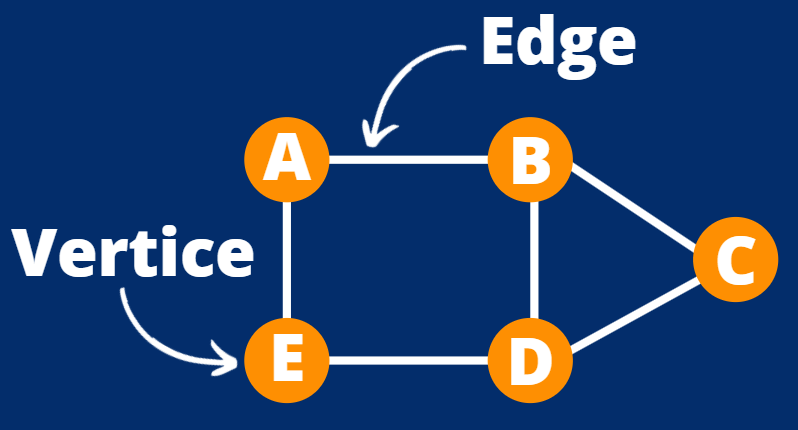Components of a Graph

It is evident from the above diagram that a graph consists of two basic components, Edges and vertices.

Vertices: Vertices or nodes are the elements whose relationships are expressed using edges.
For example: A bi-directional relationship is expressed with two nodes and an undirected edge between them.

Edges: Edges are the elements that are used to represent the connections between vertices in a graph.
For example: A one-way or two-way relationship between two nodes is expressed by an edge between them.

### Types of Nodes

Root node: In a graph, the root node is the ancestor of all other nodes. There is only one root node in each graph. In general, traversing a graph must begin at the root node.

Leaf nodes: Leaf nodes are nodes in a graph that do not have any successors. Only ancestor nodes exist in these nodes. They can have several incoming edges, but they won't have any outgoing edges.

Let’s see the various types of graphs now.

## Types of Graphs

In data structures, we use four different types of graphs. These are as follows:

### Undirected Graph :

An Undirected Graph is one in which the edges of a graph do not point in a single direction and their orientation can be classified as bi-directional.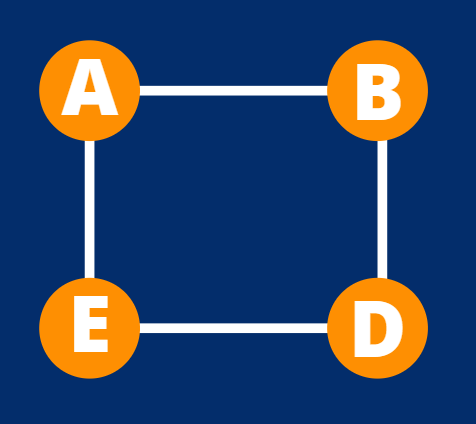Directed Graph :

The Directed graph, in contrast to the Undirected graph, contains edges that point in only one direction and can be defined as unidirectional.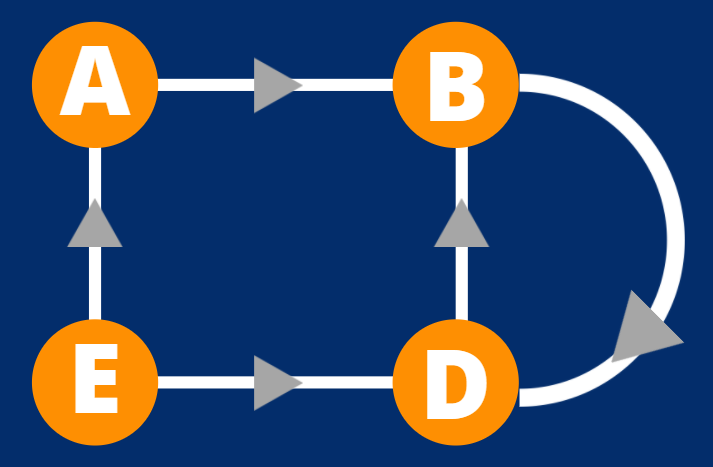Cyclic Graph :

A graph is called a Cyclic Graph when one of its paths starts at one vertex and ends at the same vertex. A cycle is the term used to describe this entire path.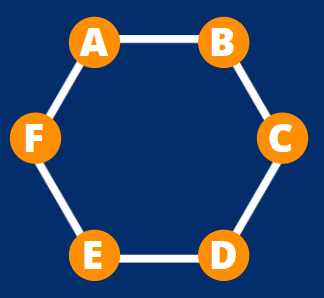Note: Acyclic Graph doesn’t have a cycle.

### Weighted Graph :

Every graph, according to our knowledge, contains certain edges, and when we assign a specific weight or cost to each edge of our graph, we call it a Weighted Graph.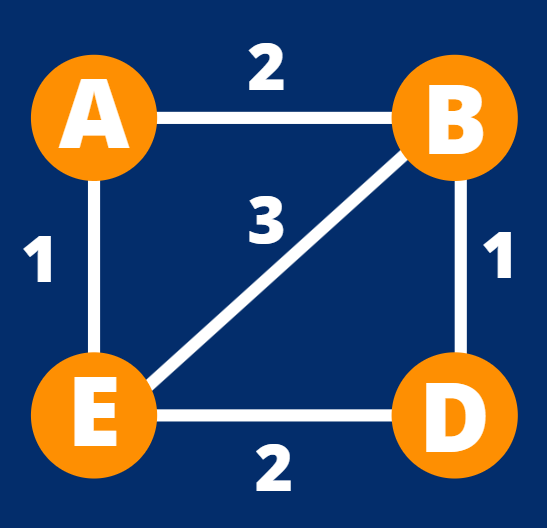So till now, we have seen the different types of graphs. But the real question here is how can we represent these graphs in our program?

## Graph Representation

There are two common ways for graph representation, and these are as follows :

A square matrix with the same number of rows and columns is called an adjacency matrix. Each matrix cell represents an edge or the connection between two nodes. This is the most used method of graph representation.

• An Adjacency Matrix consists of M*M elements where A(i,j) will have the value as 1 if the edge starts at the ith vertex and ends up at the jth vertex.
• The A(i,j) will have the value as 0 if the edge starts from a vertex and ends on the same vertex.

We can represent graphs in the following way using Adjacency Matrix :

UNDIRECTED GRAPH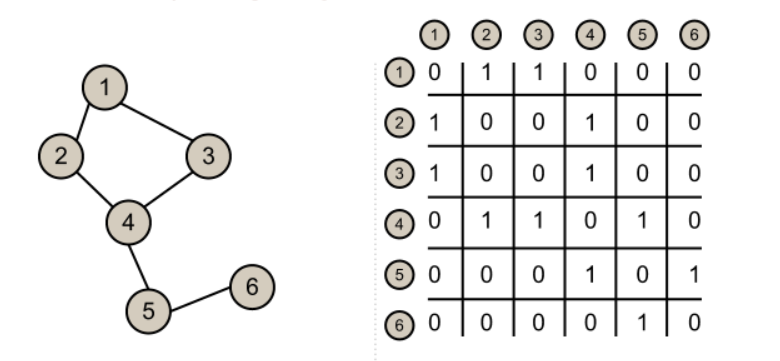DIRECTED GRAPH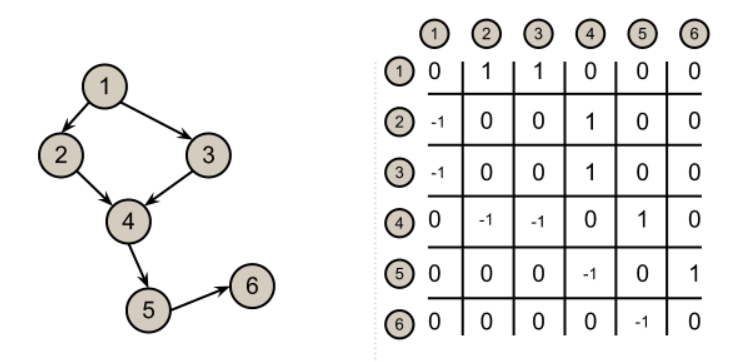Note: A sparse matrix has the majority of its elements be zero, whereas a dense matrix has the majority of its elements be non-zero.

An adjacency list is said to be an array of different lists, and every element of this array is a list of its corresponding vertice or the neighbour vertice. This is the more optimal way of graph representation.

We can say that the ith node of our adjacency list points to another list of all the vertices which are in a direct connection with the ith vertex.

Refer to the diagram below to understand the graph representation using adjacency list in directed and undirected graphs.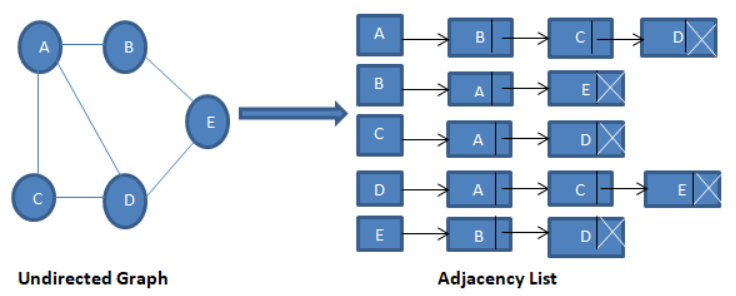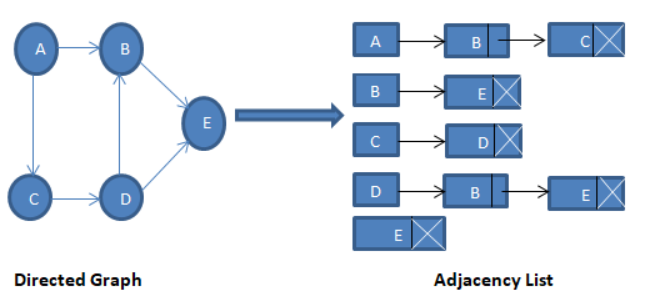You might be wondering which is better since both representations are used to depict graphs. Some of the basic differences between these two ways of graph representation is given below:

• The amount of edges (rather than the number of nodes) determines how much memory is used, which might save a lot of space if the adjacency matrix is sparse.
• Checking if a specific edge is present between any two nodes takes a little longer than adjacent matrices.
• Because we can directly access any node's neighbours, iteration over all edges is fast.
• It works faster in adding or deleting a node.

• The space needed by an adjacency matrix is O(N²), where N is the number of vertices in a graph.
• Checking if a specific edge is present between any two nodes is fast.
• It is slow in adding or deleting a node.
• Iteration over edges is slow.

Let’s see some of the frequently asked questions on this topic.

1. What is a graph?
A non-linear data structure that contains elements called nodes or vertices, which are connected to each other with the help of edges.

2. How many types of graphs do we have in Data Structures?
There are a total of 17 types of graphs in data structures.

3. How can we search elements in a graph?
We can search elements using Depth-Search and Breadth-First Search in a graph.

4. What are graphs used for?
There are various applications of graphs in computer science. Some of them include browsers, Google Maps, GPS, and social media platforms.

5. What is a cycle graph?
A cyclic graph is the one that contains a cycle or we can say that the graph has vertices that are connected in a closed direction.

## Key Takeaways

In this article, we ran you through the fundamental concepts of graph data structure. We’ve covered the various types of graphs and their representation. After reading this, you can move to the implementation of graphs.

Graph theory is an important concept of computer science, here’s an amazing article to clear your concepts. Also, if you are preparing for placements and other exams, we recommend you to go through the Top Graph Interview Questions.

Many students miss minor nuances while preparing for an interview. Therefore, one must rely on a trustworthy source to practice perfectly. Coding Ninjas has brought you various interview experiences of companies like Amazon, Google, Microsoft, and many more via its platform Code Studio. Do check it out.

Happy Learning!